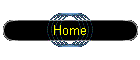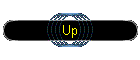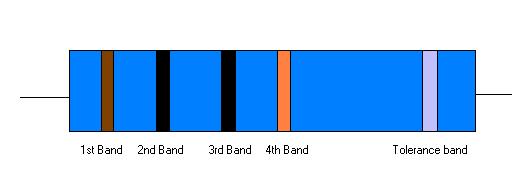Resistor colour codesfirst 3 bands on left are the values, 3rd is the multiplier, the right hand band is the tolerance. so in the above we have 1, 0, 0, 3 this is 100 with 0x3 = 100,000 = 100k Ohms resistor. (sometimes the 3rd band is omitted)   Black = 0 Brown = 1 Red = 2 Orange = 3 Yellow = 4 Green = 5 Blue = 6 Violet = 7 Grey = 8 White = 9   Tolerance bands silver = +/- 10% gold = +/- 5% ------------------------------------------